We’ve put together the best math brain teasers and number puzzles, which can be solved without even using a pen and paper.

## Tricky Math Questions With Answers

1

### What Is the Weight Brain Teaser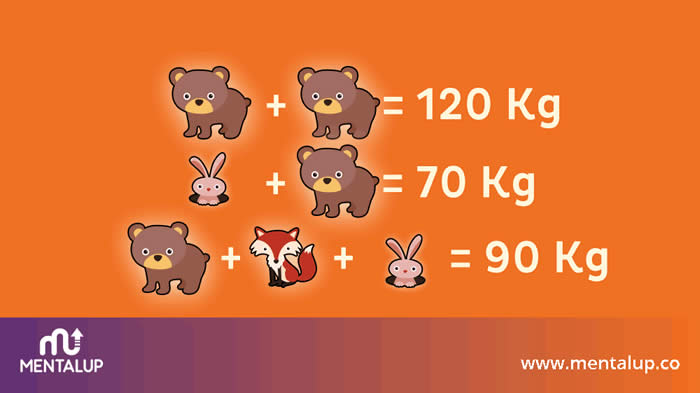What is the weight of each animal? 🐰 🛵

It’s one of the most popular math riddles these days.

If two bears are 120kg, one bear is 60kg.

A bear and a rabbit are 70kg If the bear is 60kg alone, then the rabbit is 10kg.

Since the bear and the rabbit are 70kg, the weight remaining for the fox is 20kg.

If you managed to solve this math question, then you should try our more advanced logical-mathematical brain teasers. Join MentalUP in two simple steps to compare your math performance with your peer group.

MentalUP Brain Teasers:

2

### What Number Should Replace the Question Mark?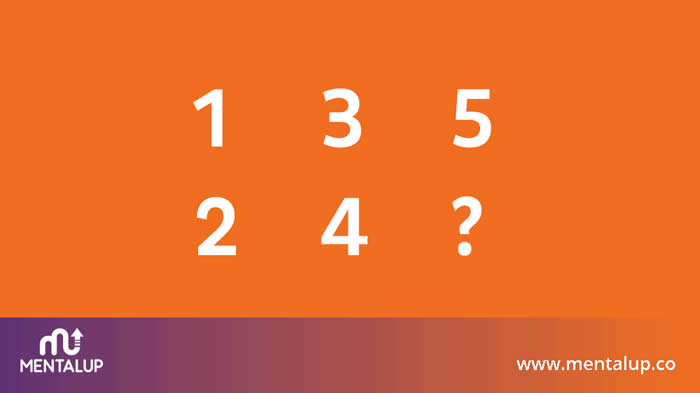This may not be a number. Think of a situation in everyday life where these numbers appear. 🧐

Let's give you a hint...

It's a lever in the car with numbers on it.

You guessed the answer now, right?

You guessed it, right?

Exactly! It’s a gear stick.

So the question mark will be replaced by the letter ‘R’.

Keep your mind fit and sharp with logical math puzzles:

3

### Tricky Math Question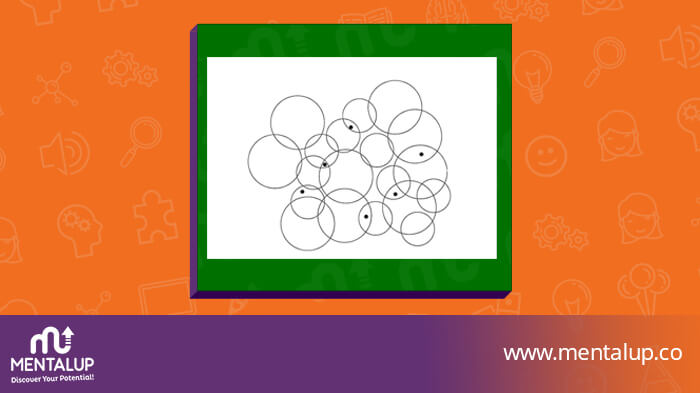Can you count the number of circles that contain a black dot?

It wasn't hard to find the answer to this math puzzle. If you look carefully at the image, you’ll see that the dots are placed in intersection of many circles.

So, The correct answer is 12.

MentalUP Brain Questions App:

4

### Logic Math Problem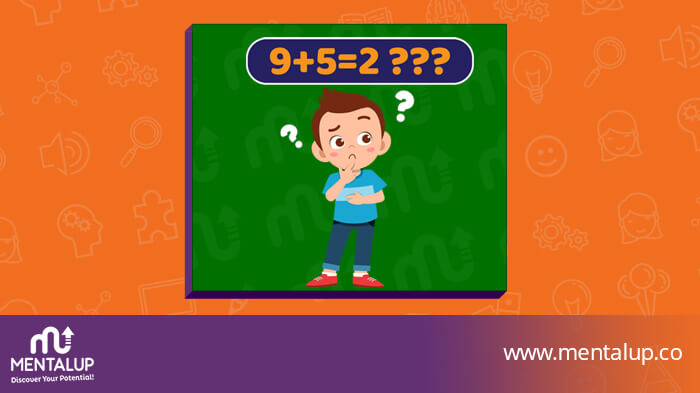I add five to nine and get two. The answer is correct, but how?

Answer: When it is 9 AM, add 5 hours to it and you will get 2 PM.

Want to solve more puzzles?

5

### 2 3 4 5 Math Riddle & Answer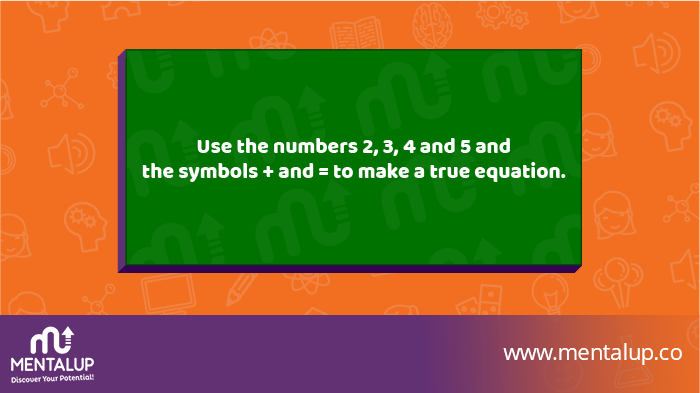Make a true equation! 🧮

It was too easy to find the answer to this math riddle!

Answer: 2 + 5 = 3 + 4

If you couldn’t find the answer don’t worry! You can make daily maths practice with MentalUP Maths Game!

6

### 8 6 13 8 Math Puzzle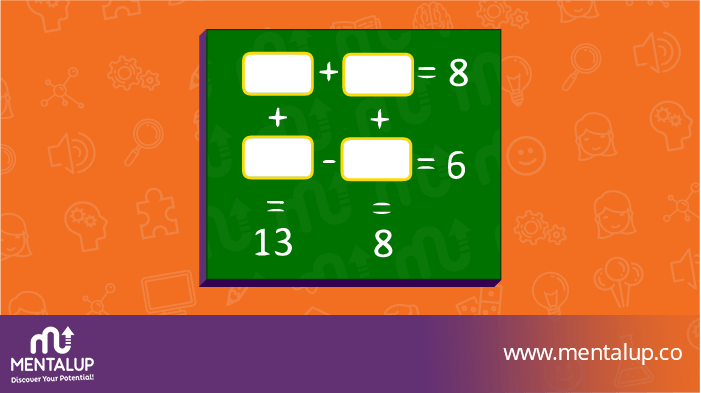Find out the numbers in the boxes! 🔢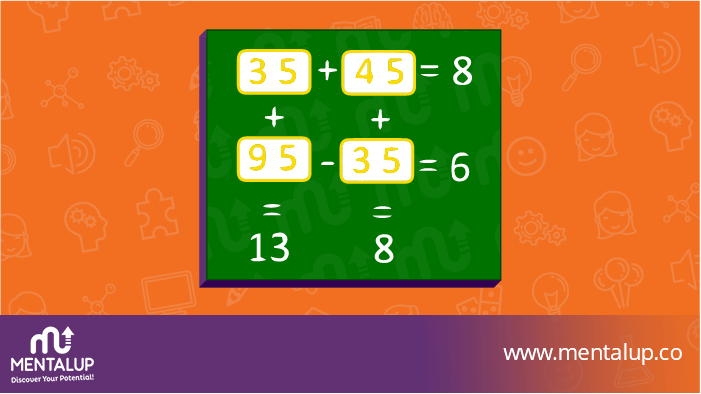We can form four equations

A+B = 8

C-D = 6

A+C = 13

B+D = 8

Let’s solve these four equations and find the variables A, B, C & D!

if we add the four equations

A+B+C-D+A+C+B+D = 8 + 6 + 13 + 8

2(A+B) + 2C = 35

2C = 35 - 2*8 (by replacing A+B)

2C = 35 - 16 = 19

C = 9.5

from 2nd Equation C - D = 6

D = C - 6

D = 9.5 - 6

D = 3.5

from 3rd equation

A+C = 13

A = 13 - C

A = 13 - 9.5

A = 3.5

from 4th equation

B + D = 8

B = 8 - D

B = 8 - 3.5

B = 4.5

## Hard Maths Puzzles With Answers

7

### Hard Math Riddle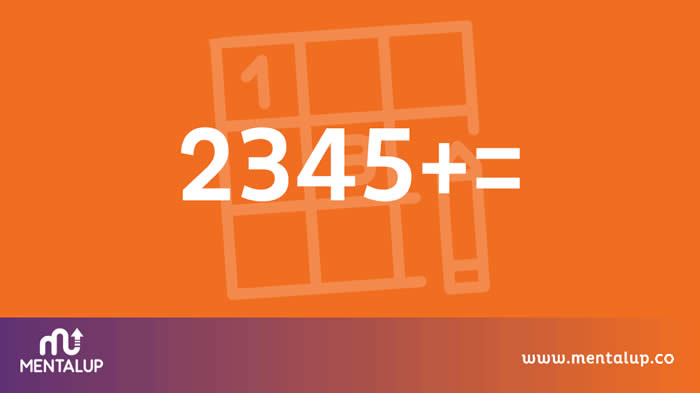Create an equation using the below numbers and mathematical symbols. ➗

Only 2 out of 10 people can solve this hard math riddle!

The answer to our question is 4+5=32

8

### Math Question Mark Brain Teaser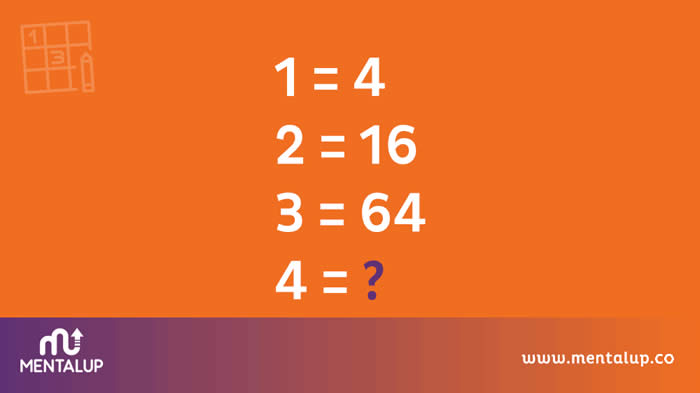What number should replace the question mark?
🤔

41=4

42=16

43=64

44= 256

Well done! Do you like it? 100’s math games and math riddles are waiting for you in MentalUP with Premium features!

9

### How Can You Get the Number 6 by Only Using Three Zeros?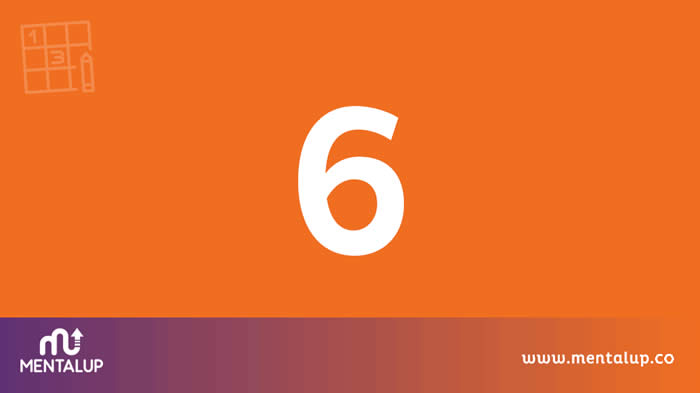One of the most sweat-inducing questions. If you don't like math riddles, we suggest you skip this question. 🧐

You need to use factorials. 0! (zero factorial) equals 1.

Here, the process is as follows: (0! +0! +0!)! = (1 + 1 + 1)! = 3! = 6

MentalUP has more math riddles for kids as well as for adults!

10

### Number Brain Teasers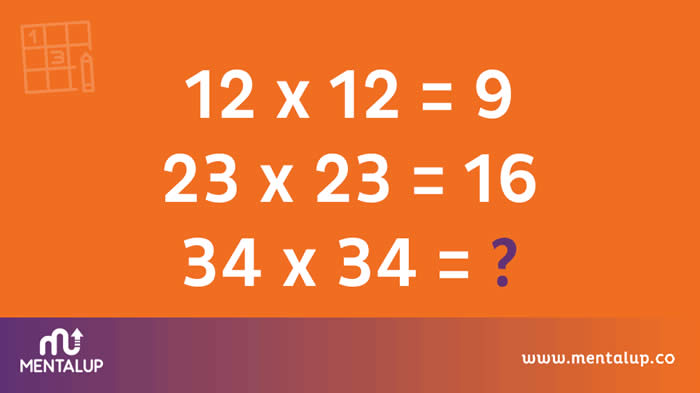What number should replace the question mark? ❓

After performing the multiplication, we reach the result by adding the individual numbers that make up the result.

If 12x12 = 144, then 1 + 4 + 4 = 9

If 23x23 = 529, then 5 + 2 + 9 = 16

If 34x34 = 1156, then 1 + 1 + 5 + 6 = 13

Couldn’t find the answer? You can always do brain exercises and improve your problem-solving skill easily with MentalUP Brain Teasers App!

11

### Hard Math Puzzle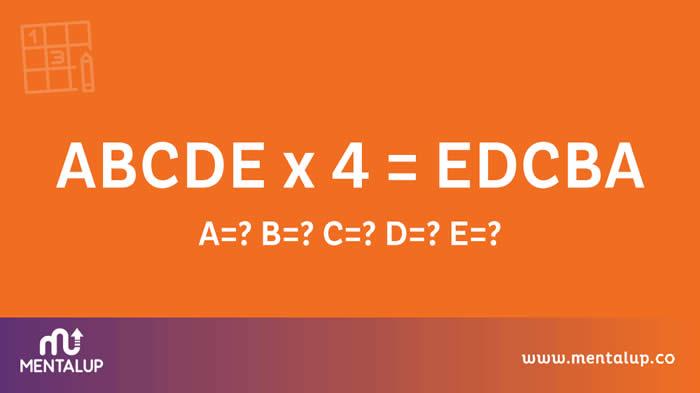If A, B, C, D and E represent different numbers, and none of them is zero, then what numbers are they? 🤓

There is a number in math that when multiplied by 4, the opposite will appear.

This number is: 21978

So A = 2, B = 1, C = 9, D = 7, E = 8

Try MentalUP for more math brain puzzle!

12

### Math Puzzles Brain Teasers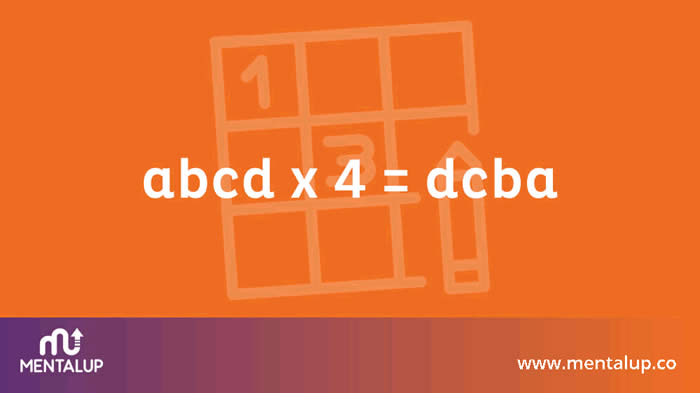Which 4-digit number multiplied by 4 gives the same number in reverse? 💡

When multiplied by 4, the number 2178 is 8712.

Math riddles is a good exercise for your thinking skills! Agree? Then, we bet you’ll like MentalUP Brain Games.

13

### Math Brain Teaser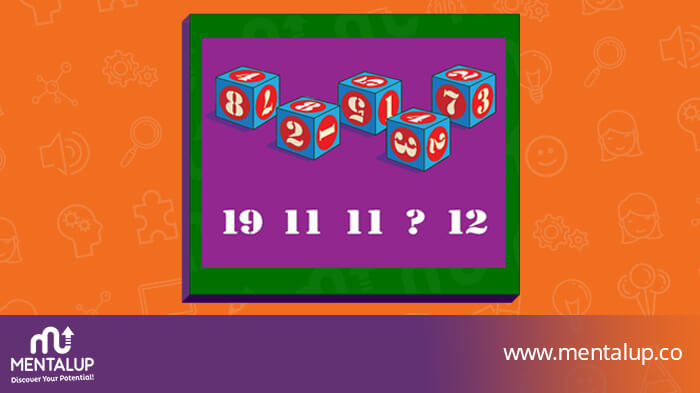Can you find the missing number? 🎲

This mysterious question mark is challenging to you! Find the answer to beat it.

The number that should replace the question mark is 9. Why? The sum of the three numbers on each die equals the number written below it.

For the first die, for example: 8 + 4 + 7 = 19; for the second: 8 + 2 + 1 = 11. Following this pattern would explain why the third die is also 11 (5 + 5 + 1) and why the mysterious question mark should be 9 (4 + 3 + 2).

14

### 5 3 2 Math Puzzle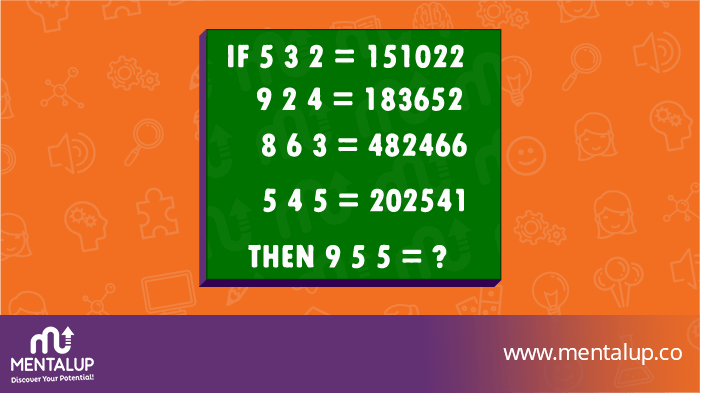Only 2 per cent of people are able to solve this math riddle. Can you be one of them? 🙄

The calculation is like this:

9 5 5 =?

9×5 = 45 (first and second)

9×5 = 45 (first and third)

Sum of 45+45 = 90 and from this deduct center no i.e. 5 = 85

Like it? We have more math riddles for you!

## Tricky Maths Puzzles With Answers

15

### How Many People Are in the Family?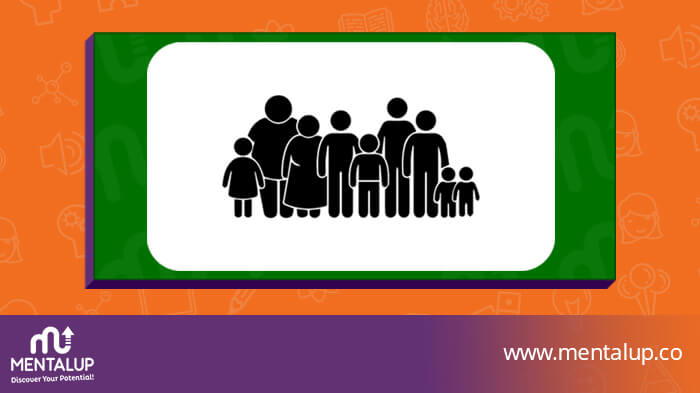Your parents have six sons including you and each son has one sister. How many people are in the family? 👨‍👩‍👦

Do you love logical maths problems? Then, it will come for you!

The answer is 9. Two parents, six sons, and one daughter.

Join MentalUP in two simple steps for more challenging math questions and brain teasers, and measure your results against your peers.

MentalUP Brain Teasers App:

16

### Funny Maths QuestionIf 3 cats can catch 3 bunnies in 3 minutes, how long will it take 100 cats to catch 100 bunnies? 😺

Attention please! It will need you as well as your math skills to find an answer to this math riddle.

It’s a tricky math riddle question to test your attention skills. It will take 3 minutes for 100 cats to catch 100 bunnies. 1 cat can catch 1 bunny in 3 minutes, so 100 catch can catch 100 bunnies in 3 minutes.

Check out MentalUP for more tricky math riddles!

17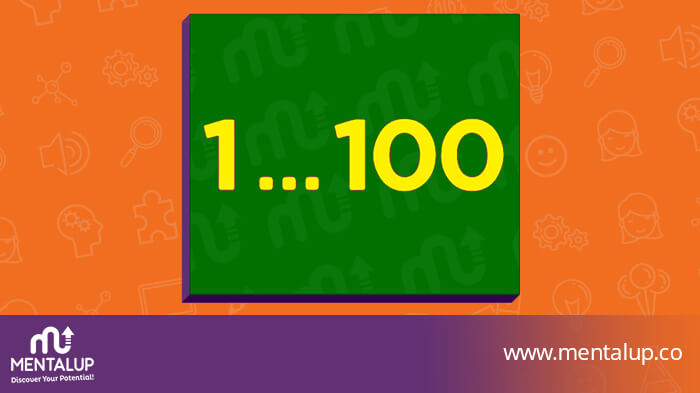How many times does the digit 5 occur in the numbers from 1 to 100? ⌚️

The answer is 20. The digit 5 appears ten times as the last digit (5, 15, 25 … 95) and ten times as a first digit (50, 51, 52 … 59).

Check out MentalUP for more fun maths brain puzzles!

18

### Funny Mathematical Picture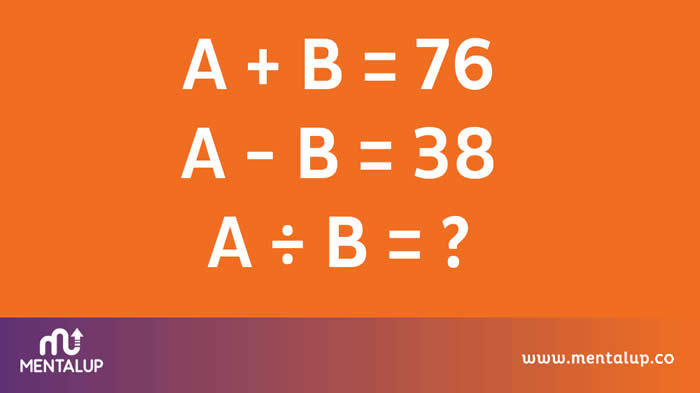What number should replace the question mark? 💡

(A+B)=76

-A+B=-38

If it is added together, 2B=38, B=19 is found.

A = 57 if B = 19.

57/19= 3

MentalUP presents users to math puzzles with unique difficulties level to develop themselves constantly.

19

### Mr Smith Has 4 Daughters Riddle & Answer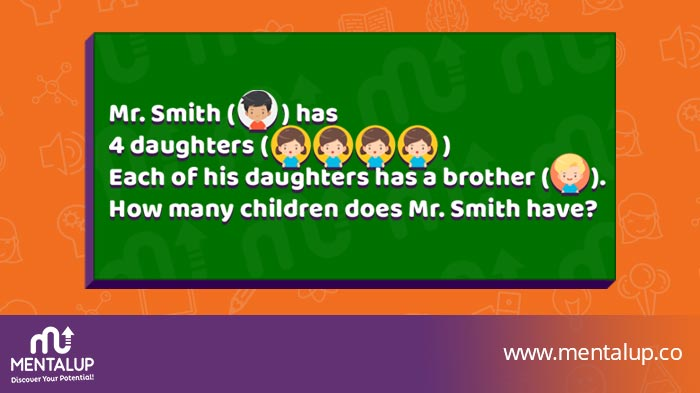Mr Smith has 4 daughters. Each of his daughters has a brother. How many children does Mr Smith have? 🧒

He has 5 children. All of the daughters have the same 1 brother.

You’re doing great! You can continue with MentalUP to access more math riddles and see your detailed performance reports:

20

### An Easy Math Puzzle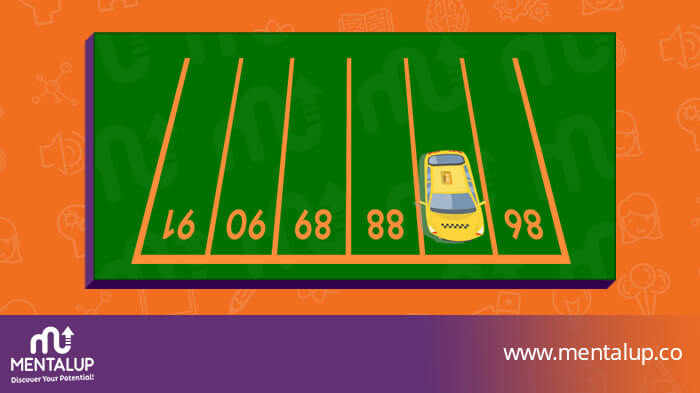What is the number of parking space containing the car? 🚘

This math question was asked to 6-years-old for and elementary school entrance exam.

Check out MentalUP for more maths brain teasers.

## Visual Math Puzzles With Answers

21

### 9 Dot Brain Teaser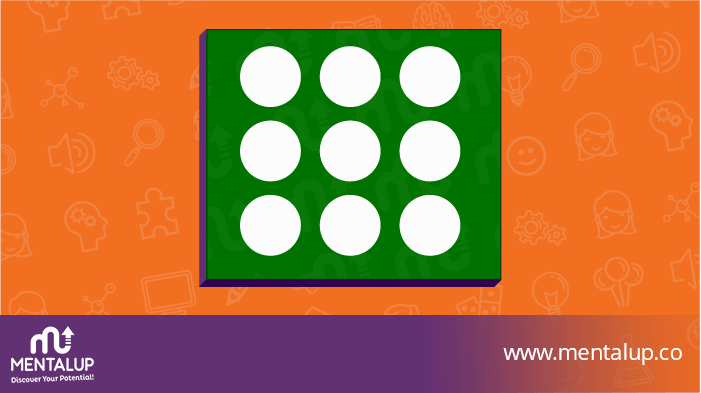Connect the dots with no more than 4 straight lines without lifting your hand from the paper. ✍️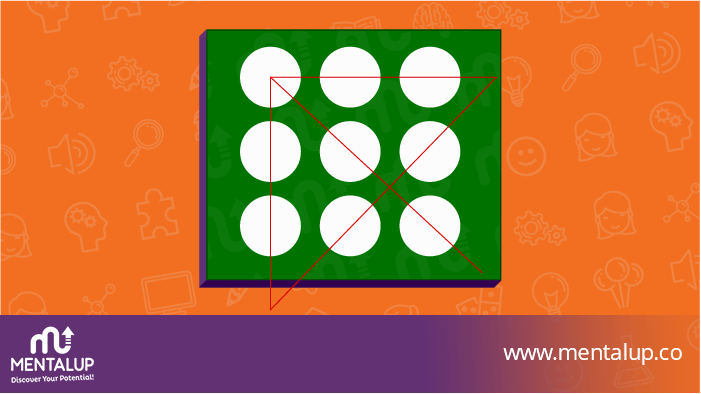22

### Take 1000 and Add 40 to It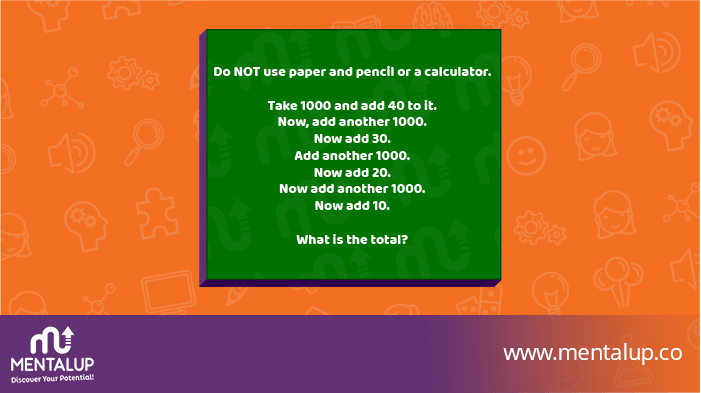‘You have 1000 add 40 riddle’ is so popular these days.⭐️

Note: This must be done in your head. It isn’t easy as you think. Try it!

If you’ve calculated it quickly, we must admit you have impressive math skills!

For the ones who cannot find this number; just calculate it by following these steps:

1000+40=1040,
1040+1000+30=2070,
2070+1000+20=3090,
3090+1000=4090,
4090+10=4100.

Check out MentalUP for more maths brain teasers

23

### Find the Car Puzzle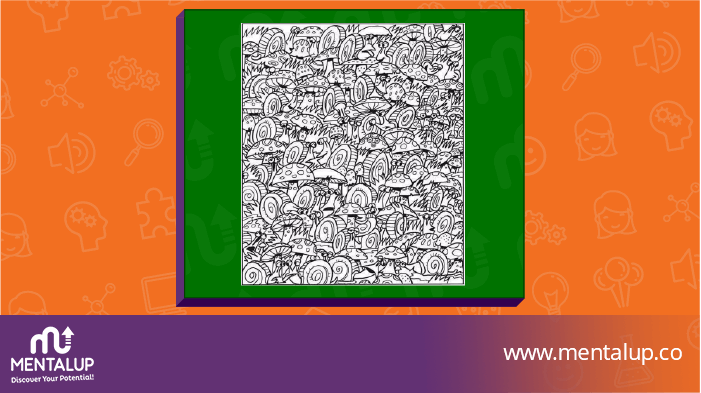Can you find the car in the picture full of snails and toadstools?

Tip: It isn’t a tricky puzzle! You can find the answer from the image.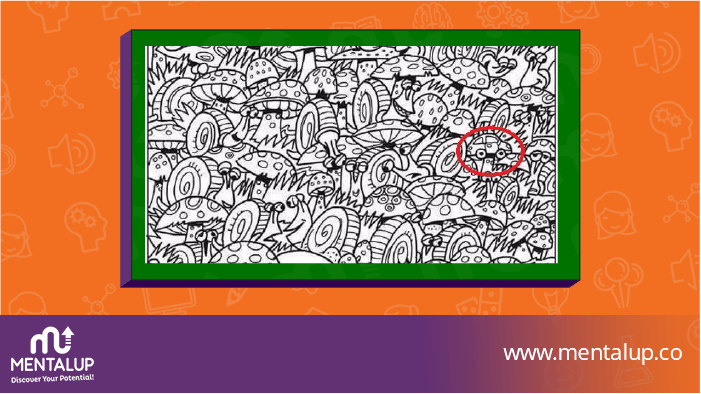24

### Maths Number Puzzle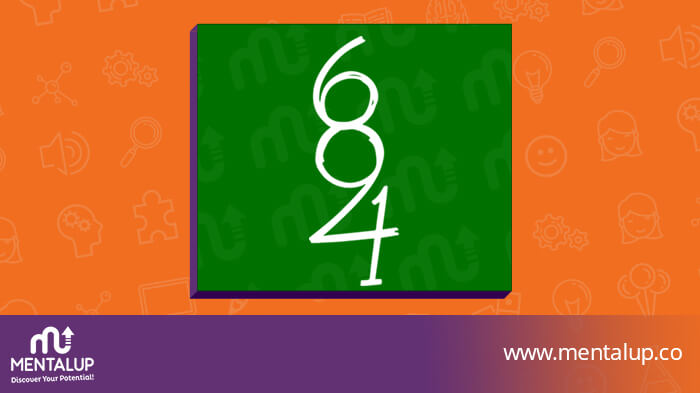How many numbers do you see here? 🔢

When you look carefully at the image, you’ll see the 0, 1, 2, 3, 4, 5, 6, 7, 8, 9.

If you’re thinking, “I trust my math logic skills and want to compare my scores with my peer group”, then join MentalUP with two simple steps!

MentalUP Brain Teasers:

## Logical Math Problems With Answers

25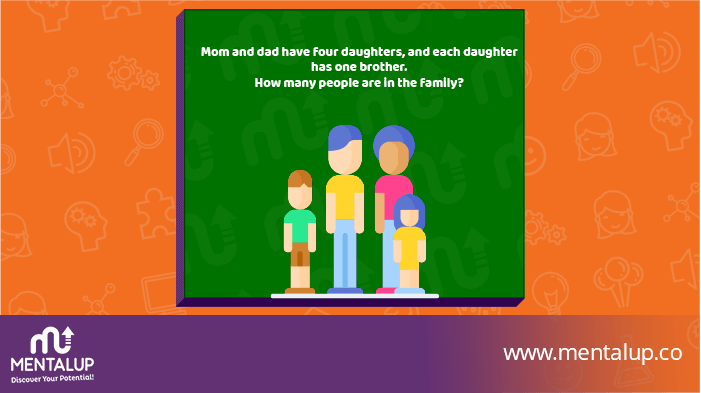Can you find out how many people are in this family? 👨‍👩‍

Tip: You need to put yourself into someone's shoes to find of answer a math riddles like that

(Dad + Mom + Four Daughters + 1 Son)

26

### The Viral 1 + 4 = 5 Puzzle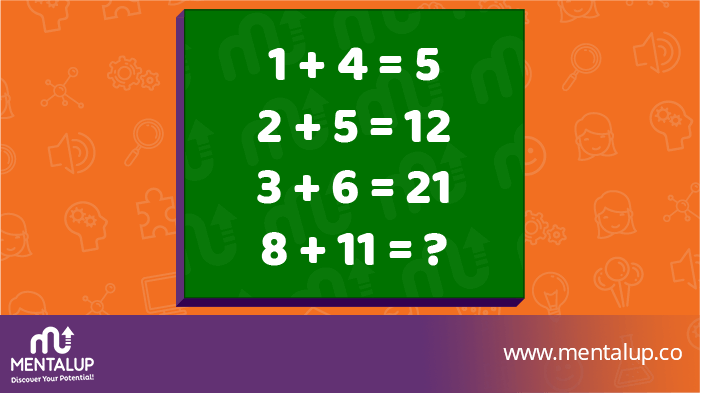Only one in a thousand will figure it out. What about you? 😅

The first equation is true, the second and third are false, and the answer to the equation should be 19.

Math puzzles like this aren’t about literally interpreting mathematical symbols. They are about identifying a pattern in the set of equations and applying it to the unknown.

So, add the first number to the product of the two numbers to get the answer.

a + b means a + ab

This works for the known equations.

1 + 4 means 1 + 1(4) = 5

2 + 5 means 2 + 2(5) = 12

3 + 6 means 3 + 3(6) = 21

Applying the pattern to the last equation gives the answer of 96.

8 + 11 means 8 + 8(11) = 96

27

### Which Tank Will Fill First Puzzle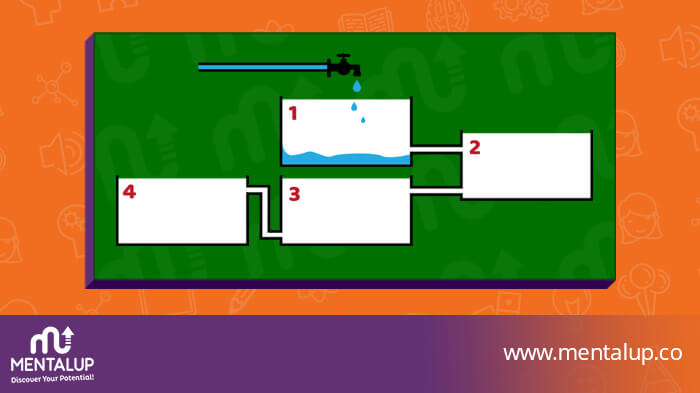It’s a hard math puzzle! Don’t worry, we think you will find the answer if you give a shot! 🚰

The first tank to fill up will be the third one, then the fourth and further the second and the first.

For more math puzzle questions, click the button and start using MentalUP:

28

### In 1990 a Person Is 15 Years Old Riddle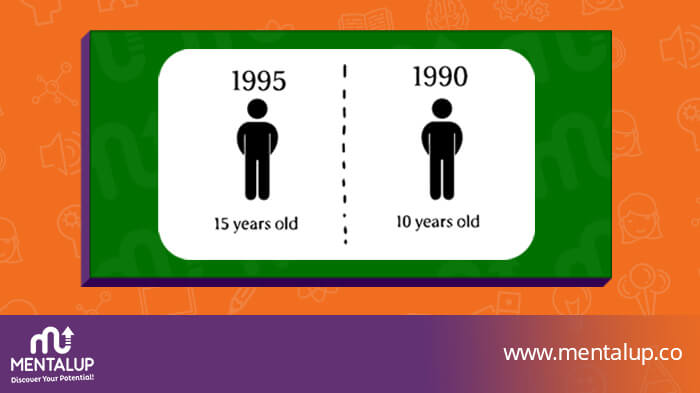In 1990, a person is 15 years old. In 1995, that same person is 10 years old. How can this be? 😮

It was a hard math riddle for kids as well as adults. The person was born in 2005 BC.

Test yourself with visual brain teasers and other areas with MentalUP:

29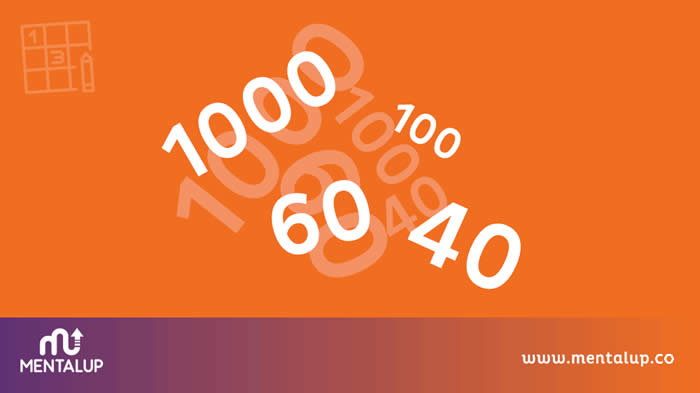What is 1000 + 40 + 1000 + 30 + 1000 + 20 + 1000 + 10? 👀

The first tank to fill up will be the third one, then the fourth and further the second and the first.

For more math puzzle questions, click the link and start using MentalUP:

30

### Guess the Missing Number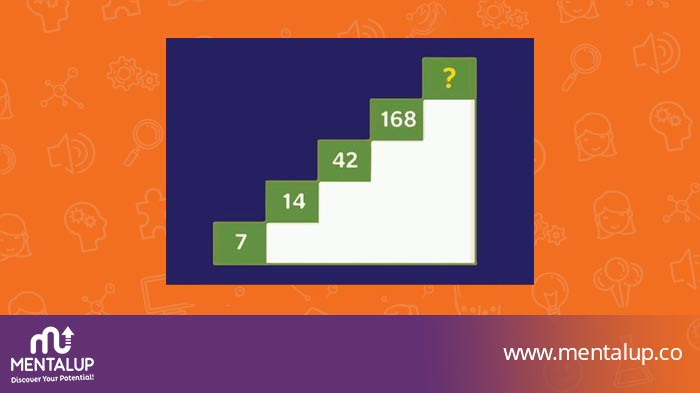Proceeding up the staircase, the numbers form a logical sequence. What number belongs on the top step? 🕵️‍

Each number is found by multiplying the number that corresponds to its step by the number that precedes it.

Need to improve your math skills? Then why don’t you make it fun and educational with using MentalUP?

Math brain teasers for adults & kids are compelling intelligence exercises. Solving such questions frequently is an excellent way to increase your mathematical intelligence and be ready for math exams. These are also very similar to question samples of Math Kangaroo Past Papers!

You will need more mathematical puzzles, math word problems, math apps, apps to improve math skills for adults and kids, or brain puzzles to improve your analytical thinking skills. Don’t worry. There is a free app that you can access hundreds of brain teasers, puzzles, math games to play at home, riddles for kids, riddles for adults, and other gamified cognitive exercises!

Don’t want to download an app? Then check it out: Brain Puzzles

YOU MIGHT ALSO LIKE: